• 本章内容：介绍了无约束和有约束两种类型的非线性优化一、无约束优化无约束优化的一般形式为: 如果要求最大值，则 1.1 fminunc() 介绍：是MATLAB求解无约束优化的主要函数，算法有：信赖域(trust region)算法和拟...
本章内容：介绍了无约束和有约束两种类型的非线性优化一、无约束优化无约束优化的一般形式为:如果要求最大值，则1.1 fminunc() 介绍：是MATLAB求解无约束优化的主要函数，算法有：信赖域(trust region)算法和拟牛顿法(quasi-Newton)，详解如下（对算法有要求的可以看看）:Unconstrained Nonlinear Optimization Algorithms​ww2.mathworks.cn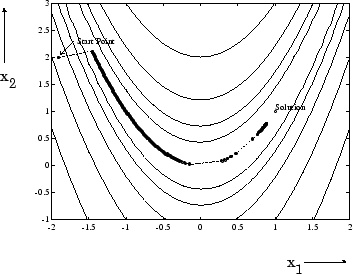例1、求Banana function的最小值（因其函数图像形似香蕉得名）一般优化算法中会用到函数的导数信息，故可以将目标函数的导数以函数的形式输入到fminunc中。若不提供导数信息，fminunc内部会用差分代替导数，由于差分方法计算出的导数值与导数函数计算出的值存在误差，所以，若能为fminunc提供目标函数的导数函数信息，更便于计算。（1）拟牛顿法函数function f = BanaFun(x)
f = 100*(x(2)-x(1)^2)^2+(1-x(1))^2;主程序options = optimoptions('fminunc','display','iter');     %显示迭代过程，由于matlab推荐采用新的设置optimoptions替代optimset，所以这里跟以前有点不同
x = [-1.9,2];                                           %初始迭代点
[x,fval,exitflag,output] = fminunc(@BanaFun,x,options)结果> In fminunc (line 395)
%迭代次数  目标函数计算次数 函数值      步长            一阶导数值
First-order
Iteration  Func-count       f(x)        Step-size       optimality
0           3           267.62                      1.23e+03
1           6          214.416    0.000813405            519
2           9          54.2992              1            331
3          15          5.90157       0.482557           1.46
4          21          5.89006             10           2.58
5          24          5.84193              1           6.56
6          36          4.10288         3.3617           15.2
7          42          4.08488       0.115159           19.9
8          48          3.39007             10           11.3
9          51          3.13363              1           20.7
10          54          2.46989              1           3.18
11          60          2.12375       0.680215           8.62
12          63          1.92978              1           8.85
13          66          1.43583              1            2.9
14          72          1.29696       0.424794            6.5
15          75          1.11139              1           5.84
16          78         0.846834              1           2.07
17          84         0.694787       0.355489           5.72
18          87         0.610241              1           3.12
19          93         0.478524       0.655536           5.39
First-order
Iteration  Func-count       f(x)        Step-size       optimality
20          96         0.343127              1           1.82
21         102          0.28558            0.5            3.9
22         105         0.194608              1           3.02
23         108         0.135004              1           2.02
24         114        0.0854278       0.431816           2.78
25         117        0.0672604              1           2.68
26         120        0.0377243              1           2.42
27         126        0.0157395       0.424018           1.72
28         129        0.0113891              1           1.66
29         132       0.00400353              1           1.11
30         138      0.000778789       0.478349          0.549
31         141      0.000349468              1          0.452
32         144      3.21971e-05              1          0.113
33         147      6.28136e-06              1         0.0865
34         150      4.74855e-08              1       0.000383

Local minimum found.                                %找到了局部最优点

Optimization completed because the size of the gradient is less than
the default value of the optimality tolerance.

<stopping criteria details>

x =                                                 %最优解

0.9998    0.9996

fval =                                              %最优函数值

4.7485e-08

exitflag =

1

output =

包含以下字段的 struct:

iterations: 34
funcCount: 150
stepsize: 0.0034
lssteplength: 1
firstorderopt: 3.8341e-04
algorithm: 'quasi-newton'               %拟牛顿法
message: 'Local minimum found.…'（2）信赖域法函数function [f,g] = BanaFun(x)
f = 100*(x(2)-x(1)^2)^2+(1-x(1))^2;
g = [100*(4*x(1)^3-4*x(1)*x(2))+2*x(1)-2;100*(2*x(2)-2*x(1)^2]); %目标函数的偏导数矩阵主程序options = optimoptions('fminunc','Algorithm','trust-region','SpecifyObjectiveGradient',true);
%注意时optimotions不是optimset
%'Algorithm','trust-region'算法选择信赖域法
%调用目标函数导数
x = [-1.9,2];
[x,fval,exitflag,output] = fminunc(@BanaFun,x,options)1.2fminsearch()函数算法：可变多面体算法例2、在例1的基础上用fminsearch()函数函数function f = BanaFun(x)
f = 100*(x(2)-x(1)^2)^2+(1-x(1))^2;主程序options = optimset('display','iter');
x = [-1.9,2];
[x,fval,exitflag,output] = fminsearch(@BanaFun,x,options)结果优化已终止:
当前的 x 满足使用 1.000000e-04 的 OPTIONS.TolX 的终止条件，
F(X) 满足使用 1.000000e-04 的 OPTIONS.TolFun 的收敛条件

x =

1.0000    1.0000

fval =

4.0686e-10

exitflag =

1

output =

包含以下字段的 struct:

iterations: 114
funcCount: 210
algorithm: 'Nelder-Mead simplex direct search'
message: '优化已终止:…'其实无约束非线性优化还有许多算法，但都讲篇幅过大，因此这里只讲几种主要的算法。二、约束最优化fmincon()是最主要的求解约束最优化的函数形式：算法：大规模内点法，SQP算法，基于内点反射信赖域算法等。约束非线性最优化算法​ww2.mathworks.cn例3、求解如下优化问题函数function f = confun(x)
f = -x(1)*x(2)*x(3);主程序options = optimoptions('fmincon','Display','iter','Algorithm','sqp');  %选用sqp算法
A = [-1,-2,-2;1,2,2];
b = [0;72];
x0 = [10,10,10];                                                        %初始迭代点
Aeq = [];
beq = [];
lb = [];
ub = [];
nonlcon = [];                                                           %对应标准形式中的ceq
[x,fval,exitflag,output] = fmincon(@confun,x0,A,b,Aeq,beq,lb,ub,nonlcon,options)结果 Iter  Func-count            Fval   Feasibility   Step Length       Norm of   First-order
step    optimality
0           4   -1.000000e+03     0.000e+00     1.000e+00     0.000e+00     1.000e+02
1           9   -1.364305e+03     0.000e+00     7.000e-01     3.340e+01     1.674e+02
2          13   -3.291805e+03     0.000e+00     1.000e+00     1.420e+01     1.673e+02
3          17   -3.437592e+03     0.000e+00     1.000e+00     8.824e+00     1.247e+02
4          21   -3.455626e+03     0.000e+00     1.000e+00     2.423e+00     3.448e+00
5          25   -3.455999e+03     0.000e+00     1.000e+00     2.942e-01     1.630e-01
6          35   -3.455999e+03     1.421e-14     1.176e-01     4.405e-03     1.687e-01
7          39   -3.456000e+03     0.000e+00     1.000e+00     2.144e-02     7.989e-02
8          43   -3.456000e+03     0.000e+00     1.000e+00     1.037e-02     1.709e-04

Local minimum found that satisfies the constraints.

Optimization completed because the objective function is non-decreasing in
feasible directions, to within the default value of the optimality tolerance,
and constraints are satisfied to within the default value of the constraint tolerance.

<stopping criteria details>

x =

24             12             12

fval =

-3456

exitflag =

1

output =

包含以下字段的 struct:

iterations: 8
funcCount: 43
algorithm: 'sqp'
message: 'Local minimum found that satisfies the con…'
constrviolation: 0
stepsize: 50/4823
lssteplength: 1
firstorderopt: 121/707851三、大规模优化问题举例例4、求解如下优化问题（含200个变量）函数function f = Fun(x)
f = 0;
n = 200'
for ii = 1:n
f = f+(x(ii)-1/ii)^2;
end主程序n = 200;
x0 = 10*ones(1,n);     %初始迭代点
options = optimoptions('fminunc','Display','iter','Algorithm','quasi-newton');
[x,fval,exitflag,output] = fminunc(@Fun,x0,options)结果fval =

1.1140e-14总结本文介绍了非线性优化算法，主要分为无约束和有约束两种情况。优化算法中最重要的是算法的选择，本文并没有对这些算法进行对比，这将在以后的章节中介绍。
展开全文• 定义 如果目标函数或约束条件中至少有一个是非线性函数时的最优化问题就叫做非线性规划问题 其它情况: 求目标函数的最大值或约束条件为小于等于零的情况都可通过取其相反数化为上述一般形式1二次规划用MATLAB软件...
• 一元无约束规划 [x, fval]= fminbnd (f,x1,x2）其中fun为目标函数，支持字符...例1 求函数在区间【0，8】上的最大值、最小值。 代码 如下 y='2*exp(-x)*sin(x)' [xmin,fmin]=fminbnd(y,0,8) %fplot(y,[0,8]) 答...
一元无约束规划

[x, fval]= fminbnd (f,x1,x2）其中fun为目标函数，支持字符串，inline函数，句柄函数，[x1,x2]为优化区间。输出x为最优解，fval为最优值。

例1 求函数在区间【0，8】上的最大值、最小值。

代码 如下

y='2*exp(-x)*sin(x)'
[xmin,fmin]=fminbnd(y,0,8)
%fplot(y,[0,8])

答案是

y =
'2*exp(-x)*sin(x)'
xmin =
3.9270
fmin =
-0.0279

也可以画图来看看最大值最小值的位置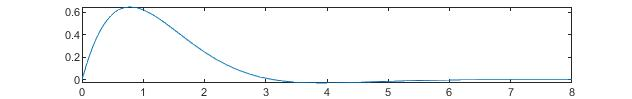求最大值的时候可以这样

y_1='-2*exp(-x)*sin(x)'
[xmax,ymax]=fminbnd(y_1,0,8)
ymax=-ymax

y_1 =
'-2*exp(-x)*sin(x)'
xmax =
0.7854
ymax =
-0.6448
ymax =
0.6448

也可以运用inline函数求解

y_2=inline('2*exp(-x)*sin(x)')
[xmax_1,ymax_1]=fminbnd(y_2,0,8)

y_2 =
内联函数:
y_2(x) = 2*exp(-x)*sin(x)
xmax_1 =
3.9270
ymax_1 =
-0.0279

答案有几分不一样，这是由各种函数的内置算法不同影响的。

当然 ，我们也可以运用句柄函数。

先写一个m文件

function f=fun1(x)
f=2*exp(-x)*sin(x);

函数也有几种调用形式

[x1,y1]=fminbnd('f',0,8)
[x2,y2]=fminbnd('f(x)',0,8)
[x3,y3]=fminbnd(@f,0,8)

运行结果是相同的

多元无约束优化

[x, fval]= fminunc(fun,x0)

[x, fval]= fminsearch(fun,x0)

其中：输入参数fun为目标函数，支持字符串，inline函数、句柄函数，x0为初值，必须得有

注意：fminunc，fminsearch只支持函数fun自变量单变量符号，如x(1),x(2)等

例2 求解的最小值

f='100*(x(1)-x(2)^2)^2+(1-x(2))^2'
[x,fval]=fminunc(f,[0,0])
[x_1,fval_1]=fminsearch(f,[0,0])


答案为


x =
1.0000    1.0000
fval
1.9474e-11
x_1 =
1.0000    1.0000
fval_1 =
3.6862e-10

有约束优化

[x, fval]= fmincon(fun,x0,A,b,Aeq,beq,lb,ub,nonlcon)

参数解释

fun为目标函数，支持字符串、inline函数，句柄函数，x0初值，A线性不等式约束系数、b线性不等式约束常数项、Aeq线性等式约束系数，线性beq等式约束常数，nonlcon非线性约束，支持句柄函数。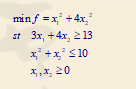先创建两个句柄函数

function f1=fun(x)
f1=x(1)^2+4*x(2)^2;

function [c,ceq]=fun(x)
c=x(1)^2+x(2)^2-10;
ceq=0;

x0=[10,10];
A=[-3,-4];b=-13;
lb=[0,0];
[x,f]=fmincon(@f1,x0,A,b,[],[],lb,[],@f3)

答案如下

x =

2.9991    1.0007

f =

13.0000

再看一个例子

例 4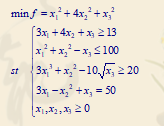先构造两个句柄函数

function f2=f(x)
f2=x(1)^2+4*x(2)^2+x(3)^2;

function [c,ceq]=fun(x)
c=[x(1)^2+x(2)^2-x(3)-100,-3*x(1)^3-x(2)^2+10*x(3)^0.5+20];
ceq=3*x(1)-x(2)^2+x(3)-50;%当非线性不等式约束有多个的时候，就要用矩阵写出约束条件。

代码

x0=[1,1,1];
A=[-3,-4,-1];b=13;
lb=[0,0,0];
[x,f]=fmincon(@f2,x0,A,b,[],[],lb,[],@f4)


x =

10.8390    0.0005   17.4831

f =

423.1423



展开全文• 首先从简单的开始，没有约束的非线性，然后就是非线性中比较特殊的一个例子，多项式的非线性，如下图 思路：用matlab求解f的hessian矩阵，然后求解hessian矩阵的特征向量，如果都大于0，就是正定，如果是大于等于...
参考 https://www.cnblogs.com/always-fight/p/9377554.html
1、首先从简单的开始，没有约束的非线性，然后就是非线性中比较特殊的一个例子，多项式的非线性，如下图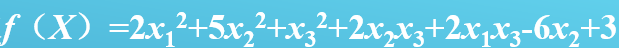思路：用matlab求解f的hessian矩阵，然后求解hessian矩阵的特征向量，如果都大于0，就是正定，如果是大于等于0，那么就是半正定，如果有小于0的，那就是非正定。前提：是求解最小值啊，如果求解最大值，那就要反过来的，不过一般都把目标函数倒过来就是了。
2、那么要找一个非正定的，来试试，看下图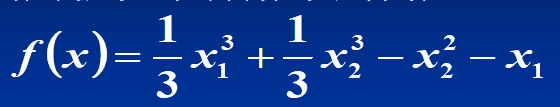用上面的思路，求解一遍看看，可以看到hessian得到的，是带有变量的，因此肯定是不定的，如下所示：
h =
[ 2x1,        0]
[    0, 2x2 - 2]
用eig求解，得到如下：
e =
2x2 - 2
2x1
那么可以细化一下，比如[0,1]这个解，是半正定的，应该是极小值，[1,1]这个解，是正定的，也是极小值，[-1,0]这个解，是负定的，应该是极大值，[-1,1]这个解，是不定的，不是极值点。所以，这几个点有的是极值点，有的不是极值点。
因此，这个肯定没有全局最优解的，对不对呢？不知道，反正，上面的这个例子肯定没有最小值。
引出一个话题：
凸函数肯定有全局最优解，即极小值就是最优解；那么如果是非凸函数，是不是就一定没有全局最优解呢？等待解决中。。。


展开全文• 定义：如果目标函数或约束条件中至少有一个是非线性函数时的最优化问题就叫做非线性规划问题． 其它情况: 求目标函数的最大值或约束条件为小于等于零的情况，都可通过取其相反数化为上述一般形式． （1）二次规划 ...
1.概念
定义：如果目标函数或约束条件中至少有一个是非线性函数时的最优化问题就叫做非线性规划问题．
其它情况: 求目标函数的最大值或约束条件为小于等于零的情况，都可通过取其相反数化为上述一般形式．
（1）二次规划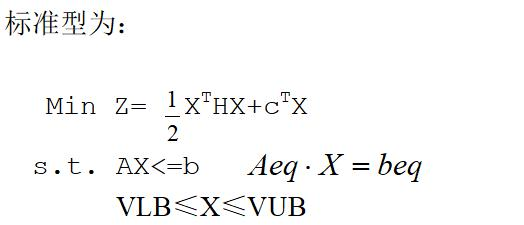用MATLAB软件求解,其输入格式如下:

x=quadprog(H,C,A,b);
x=quadprog(H,C,A,b,Aeq,beq);
x=quadprog(H,C,A,b,Aeq,beq,VLB,VUB);
x=quadprog(H,C,A,b, Aeq,beq ,VLB,VUB,X0);
x=quadprog(H,C,A,b, Aeq,beq ,VLB,VUB,X0,options);
[x,fval]=quadprog(…);
[x,fval,exitflag]=quaprog(…);
实例：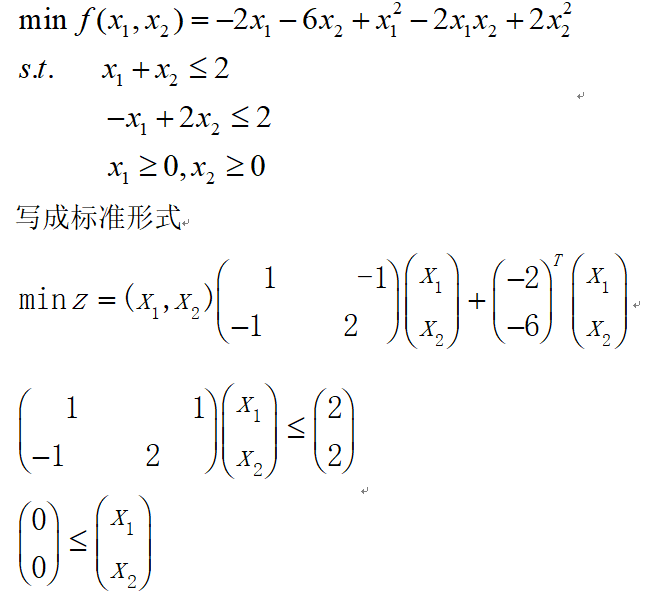Matlab命令

H=[1 -1; -1 2];
c=[-2 ;-6];
A=[1 1; -1 2];
b=[2;2];
Aeq=[];
beq=[];
VLB=[0;0];
VUB=[];
[x,z]=quadprog(H,c,A,b,Aeq,beq,VLB,VUB)

运算结果为：x =0.6667  1.3333   z = -8.2222
（2）一般非线性规划
标准型为：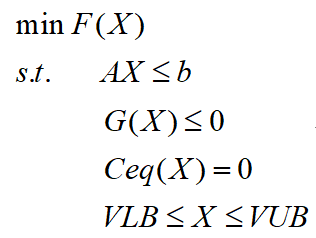其中X为n维变元向量，G(X)与Ceq(X)均为非线性函数组成的向量，其它变量的含义与线性规划、二次规划中相同.
非线性规划求解的函数是fmincon,命令的基本格式如下：
	x=fmincon(‘fun’,X0,A,b)
	x=fmincon(‘fun’,X0,A,b,Aeq,beq)
	x=fmincon(‘fun’,X0,A,b, Aeq,beq,VLB,VUB)
	x=fmincon(‘fun’,X0,A,b,Aeq,beq,VLB,VUB,’nonlcon’)
	x=fmincon(‘fun’,X0,A,b,Aeq,beq,VLB,VUB,’nonlcon’,options)
	[x,fval]= fmincon(…)
	[x,fval,exitflag]= fmincon(…)
	[x,fval,exitflag,output]= fmincon(…)
1.fun为目标函数
2.x0为初始值
3.A是不等式约束AX<=b的系数矩阵
4.b是不等式约束AX<=b的常数项
4.Aeq是等式约束AeqX=beq的系数矩阵，
5.beq是等式约束AeqX=beq的常数项，
6.lb是X的下限，
7.ub是X的上限，
8.nonlcon为非线性不等式约束
9.option为设置fmincon的参数
注意：
fmincon函数提供了大型优化算法和中型优化算法。默认时，若在fun函数中提供了梯度（options参数的GradObj设置为’on’），并且只有上下界存在或只有等式约束，fmincon函数将选择大型算法。当既有等式约束又有梯度约束时，使用中型算法。
fmincon函数的中型算法使用的是序列二次规划法。在每一步迭代中求解二次规划子问题，并用BFGS法更新拉格朗日Hessian矩阵。
fmincon函数可能会给出局部最优解，这与初值X0的选取有关。
实例2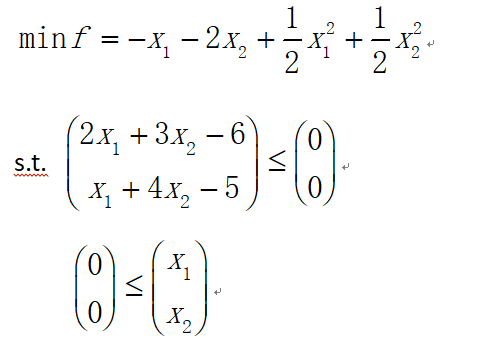先建立M-文件 ex2.m:
function f=ex2(x);
f=-x(1)-2*x(2)+(1/2)*x(1)^2+(1/2)*x(2)^2
再建立主程序main2.m：
x0=[1;1];
A=[2 3 ;1 4]; b=[6;5];
Aeq=[];beq=[];
VLB=[0;0]; VUB=[];
[x,fval]=fmincon('ex2',x0,A,b,Aeq,beq,VLB,VUB)
运算结果为：   x = 0.7647       1.0588   fval =   -2.0294

实例3：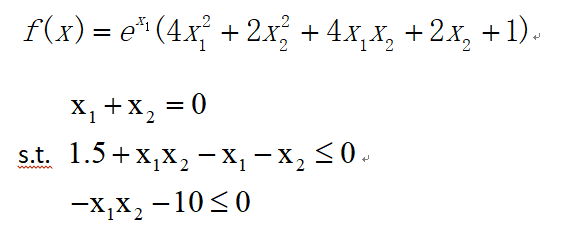先建立M文件 ex3.m,定义目标函数:
function f=ex3(x);
f=exp(x(1)) *(4*x(1)^2+2*x(2)^2+4*x(1)*x(2)+2*x(2)+1);
再建立M文件ex31.m定义非线性约束：
function [g,ceq]=ex31(x)
g=[x(1)+x(2);1.5+x(1)*x(2)-x(1)-x(2);-x(1)*x(2)-10];

主程序main3.m为:
x0=[-1;1];
A=[];b=[];
Aeq=[1 1];beq=;
vlb=[];vub=[];
[x,fval]=fmincon('ex3',x0,A,b,Aeq,beq,vlb,vub,'ex31')
运算结果为：
x = -1.2250    1.2250       fval = 1.8951

实例4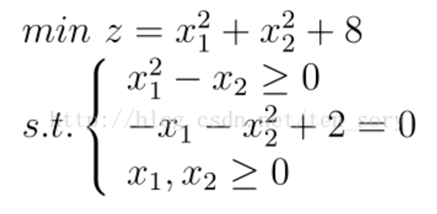function f=ex4(x)
f=x(1)^2+x(2)^2+10;

function [g,h]=ex40(x)
g=-x(1)^2+x(2);
h=-x(1)-x(2)^2+5;    %约束等式
options=optimset;
[x,y]=fmincon('ex4',rand(2,1),[],[],[],[],zeros(2,1),[],'ex40',options)
输出结果：
x = 1.3794   1.9028     y =   15.5234



展开全文算法 矩阵 动态规划
• 上一章的模型是无约束优化，即没有约束条件，只需要求目标函数的最大值或最小值。这种问题是非常简单的，只需要微积分的知识就可以求解。而这一章讲的是数学规划问题，如线性规划、非线性规划、整数规划等，需...
• 对函数取相反数而变成求最小值问题，最后把函数值取反即为函数的最大值。 使用格式如下 1.必须预先把函数存入到一个程序中,（所编的程序一定是只有一个参数， 则当为多元函数时，则x(1),x(2),x(3)… 分别代表每个...
• 非线性回归为例，目前世界上在该领域最有名的软件工具包诸如OriginPro，Matlab，SAS，SPSS，DataFit，GraphPad，TableCurve2D，TableCurve3D等，均需用户提供适当的参数初始以便计算能够收敛并找到最优解。...
• 9.2 约束优化问题 约束优化问题就是在有约束条件下寻求目标函数的最小值或最大值本节主要利用MATLAB中的函数求解线性规划非线性规划整数规划和二次规划等问题 9.2.1 线性规划问题 线性规划问题是目标函数和约束条件...
• 虽然光滑逼近会带来数值问题，例如当函数接近原始数集中的最大值时，利用凝聚函数会给优化问题增加非线性程度。尽管如此，凝聚函数被广泛应用于结构优化中，因为它是目前能够简化的优化问题中的大规模优化约束，提高...
• 遗传算法提供了一种求解非线性、多模型、多目标等复杂系统优化问题的通用框架。 先从例子开始，慢慢再总结理论。。。 【例】利用遗传算法计算函数f(x)=x*cos(5*pi*x)+3.5在区间[-1,2.5]上的最大值 先在工作区建立...
• 资源提供了遗传算法（GA）的matlab的代码实现，其中按照遗传算法流程图，代码结构按照流程顺序，被划分为不同的独立模块，代码中以求非线性函数最大值为例，通过修改此代码可实现常见的优化问题求解。
• 非线性方程与最优化问题 常微分方程的数值求解 稀疏矩阵 数据统计与分析 最大值最小值 max(x) min(x) //返回矩阵的最大元素和最小元素 [y,u] = max(a) // 返回俩个行向量，y记录a的每列的最大元素，u记录每列最大...数据处理
• 第6章 MATLAB数值计算 6.1 数据处理与多项式计算 6.2 数值微积分 6.3 离散傅立叶变换 6.4 线性方程组求解 6.5 非线性方程与最优化问题求解 6.6 常微分方程的数值求解 6.7 稀疏矩阵 6.1 数据处理与多项式计算 6.1.1 ...
• 梯度下降是迭代法的一种,可以用于求解最小二乘问题(线性和非线性都可以)。在求解机器学习算法的模型参数，即无约束优化问题时，梯度下降（Gradient Descent）是最常采用的方法之一，另一种常用的方法是最小二乘法。...梯度下降
• 第6章 MATLAB数值计算 6.1 数据处理与多项式计算 6.2 数值微积分 6.3 离散傅立叶变换 6.4 线性方程组求解 6.5 非线性方程与最优化问题求解 6.6 常微分方程的数值求解 6.7 稀疏矩阵 6.1 数据处理与多项式计算 6.1.1 ...
• 根据遗传算法和BP神经网络理论，在MATLAB软件中编程实现基于遗传算法优化的BP神经网络非线性系统拟合算法。 第4章 神经网络遗传算法函数极值寻优——非线性函数极值寻优36 对于未知的非线性函数，仅通过函数的输入...
• 非线性回归为例，目前世界上在该领域最有名的软件工具包诸如 Matlab, OriginPro, SAS, SPSS,DataFit, GraphPad 等，均需用户提供适当的参数初始以便计算能够收敛并找到最优解。如果设定的参数初始不当则计算...
• 当知道信号的相位时，可以将信号的频率直接识别为具有最大方差的频率，从而导致可以通过粒子群优化（PSO）算法解决的非线性优化问题。 当相位未知时，将将pi / 2相移方法与PSO算法集成在一起使用。 结果表明，该...
• 10．3．3 约束非线性规划问题的应用 10．4 二次规划 10．4．1 二次规划函数quadprog 10．4．2 二次规划问题的应用 10．5 有约束最小化 10．5．1 有约束最小化函数fmincon 10．5．2 有约束最小化应用 10．6 目标规划 ...
• 10．3．3 约束非线性规划问题的应用 10．4 二次规划 10．4．1 二次规划函数quadprog 10．4．2 二次规划问题的应用 10．5 有约束最小化 10．5．1 有约束最小化函数fmincon 10．5．2 有约束最小化应用 10．6 目标规划 ...
• 非线性回归为例，目前世界上在该领域最有名的软件工具包诸如OriginPro，Matlab，SAS，SPSS，DataFit，GraphPad，TableCurve2D，TableCurve3D等，均需用户提供适当的参数初始以便计算能够收敛并找到最优解。...
• 非线性回归为例，目前世界上在该领域最有名的软件包诸如Matlab, OriginPro, SAS, SPSS, DataFit, GraphPad等，均需用户提供适当的参数初始以便计算能够收敛并找到最优解。如果设定的参数初始不当则计算难以...
• 非线性回归为例，目前世界上在该领域最有名的软件工具包诸如OriginPro，Matlab，SAS，SPSS，DataFit，GraphPad，TableCurve2D，TableCurve3D等，均需用户提供适当的参数初始以便计算能够收敛并找到最优解。...1stopt
• 非线性回归为例，目前世界上在该领域最有名的 软件工具包诸如OriginPro，Matlab，SAS，SPSS，DataFit，GraphPad，TableCurve2D，TableCurve3D等，均需用户提供适当的参数初始以便计算能够收敛并找到最优解。...
• 可以是最大值-最小值，也可以是这个feature对应的数据的标准差 实现代码： # 归一化feature def featureNormaliza(X): X_norm = np.array(X) #将X转化为numpy数组对象，才可以进行矩阵的运算 #定义所需变量 mu ...# matlab非线性优化最大值matlab 订阅